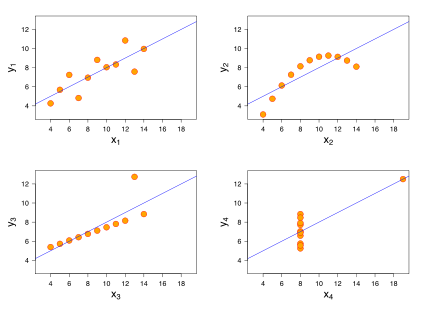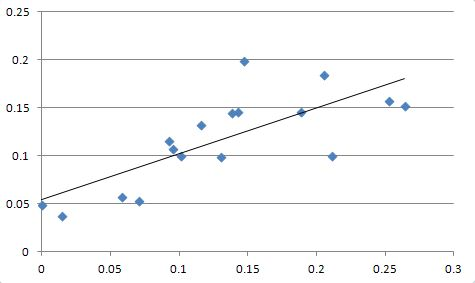# How to Perform Linear Regression in Excel

These instructions read better in this Linear Regression Excel Directions PDF file

# Introduction

Linear regression is a statistical technique used to observe trends, determine correlation, and predict future observations. The underlying principle of this technique is called the least-squared, which is the process of minimizing the distance between the predicted value of an observation and the actual value of that observation. This document provides a step-by-step guide for creating a multivariate linear regression model using Excel.

# Directions

1. Download this baseball data set in Excel format
2. You’ll see six variables in the opened Excel file.
• [X1] batting average
• [X2] runs scored/times at bat
• [X3] doubles/times at bat
• [X4] triples/times at bat
• [X5] home runs/times at bat
• [X6] strike outs/times at bat
3. If Analysis ToolPak add-in is disabled in Excel (2007), you’ll need to activate it by following these steps:
• Click the office button to open the menu options
• Click on Excel options
• Click on Add-Ins
• Under Manage, select Excel Add-ins and hit Go
• If unchecked, check Analysis ToolPak and hit OK
4. By giving names to ranges we can minimize errors and analysis would be easier. Let’s give names to our ranges by following these steps.
• Select range A2 to E29
• In the name box (in the left top corner by the formula bar), type IndependentTraining.
• Select range F2 to F29
• In the name box, type DependentTraining
• Select range A30 to E46
• In the name box type IndependentTest
• Select range F30 to F46
• In the name box, type DependentTest
• Select range A2 to E46
• In the name box, type IndependentAll
5. From the top menu, under Data, click on Data Analysis
6. From the Data Analysis options, select Regression and hit OK
7. In the Regression options box, type DependentTraining in the Input Y Range: input box and type IndependentTraining in the Input X Range: input box. Keep all the other default selections, including New Worksheet Ply option as shown in this image and hit OK
8. In the newly generated sheet, you’ll see various regression statistics. The first few in this list are Multiple R and R Square, which are measures of fit i.e. how well the regression model can explain the independent variable given all the dependent variables and observations. For more information watch this video by Khan Academy. Usually, an $$R^2$$ value close to 1 denotes that the regression model fits the given data very well and similarly, a value close to 0 denotes that the regression model doesn’t fit the given data very well. In this example, on the training data set, we obtained an $$R^2$$ of 0.53 which tells us that the regression model fits the data, but doesn’t explain all the variability.
9. Some other things of interest on this sheet are the coefficients of the input or dependent variables. These coefficients, along with the intercept, give us the regression line equation, which in this example is:
$\widehat{X6} = 0.302 -1.062*X1 -0.08*X2 + 2.086*X3 -0.76*X4 + 0.771*X5$
10. Using this equation, we can now predict the value of X6, our dependent variable, which in statistical terms is noted as $$\widehat{X6}$$. For example, row number 1 has these values:
X1 = 0.283; X2 = 0.144; X3 = 0.049; X4 = 0.012; X5 = 0.013. Let’s plug these numbers in our equation:
$\widehat{X6} = 0.302 – 1.062 \times 0.283 – 0.08 \times 0.144 + 2.086 \times 0.049 – 0.76 \times 0.012 + 0.771 \times 0.013$ $\widehat{X6} = 0.093$ The predicted X6 value of 0.093 is actually pretty close to the actual X6 value of 0.086.
11. Let’s predict all the values now. We can do it two ways:
• By typing the above equation in a cell and dragging the formula down:
• In the data sheet, in cell G1 type: PredictedX6Method1
• In cell G2, enter this formula: =0.302 -1.062*A2 -0.08*B2 +2.086*C2 -0.76*D2 +0.771*E2
• To drag this formula all the way down, click on cell G2, hover your mouse on the right-bottom corner until you see a thick plus sign, then double-click. This will predict values for X6 for all rows.
• By using the TREND function:
• In cell H1 type: PredictedX6Method2
• Select range H2 to H46
• Hit F2 key on your keyboard to enter this formula by hitting Ctrl, Shift and Enter at the same time in cell H2: =TREND(DependentTraining,IndependentTraining,IndependentAll)

Since this is an array function, it must be entered by Ctrl, Shift, and Enter keys

• It is very important to test the model on the hold-out set or the test set. We had saved rows 30 to 46 for testing and we have predictions for those observations as well.
• Select range F30 to G46
• From the main menu toolbar, click on Insert and then Scatter plot and select the first scatter plot type from the grid.
• If the predictions are very close to the actual values, we should see all the points on a 45 degree angle line. To test this, right-click on the plot and click on Add Trendline
• In the Format Trendline options box, accept all the default values and hit Close. You’ll see a straight line passing through all the data points. If the predictions were close, the trendline will start where x and y axes meet and will be in a 45 degree angle.Predicted vs Actual Trendline

• In this example, the line has a slope that may look like a 45 degree angle angle, but has an intercept of 0.05, meaning the line does not begin at 0. Therefore, we can conclude that the predictions are somewhat close to the actual observations, but they are hardly accurate.

# Notes

This tutorial, of course, doesn’t go in all the details of linear regression, all the output in Excel, and other statistics principles – this is an introduction to this topic – users who want to get started with linear regression in Excel will find this document useful. There are quite a few resources available online and in print form that provide detailed knowledge on this topic. These following are very good starting points:

#### About the Author

The author of Tableau Data Visualization Cookbook and an award winning keynote speaker, Ashutosh R. Nandeshwar is one of the few analytics professionals in the higher education industry who has developed analytical solutions for all stages of the student life cycle (from recruitment to giving). He enjoys speaking about the power of data, as well as ranting about data professionals who chase after “interesting” things. He earned his PhD/MS from West Virginia University and his BEng from Nagpur University, all in industrial engineering. Currently, he is leading the data science, reporting, and prospect development efforts at the University of Southern California.